# Density topology

(diff) ← Older revision | Latest revision (diff) | Newer revision → (diff)

The density topology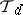onis the family of all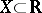with the property that everyis a density point of, i.e., such thatwhere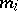stands for the Lebesgue inner measure (cf. Lebesgue measure).

The density topology was first defined in 1952 by O. Haupt and Ch. Pauc [a7], although its study did not start until 1961, when it was rediscovered by C. Goffman and D. Waterman [a5]. In both cases it was introduced to show that the classof approximately continuous functions (cf. Approximate continuity) coincides with the class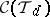of all real functions that are continuous with respect to the density topology on the domain and the natural topology on the range. Thus, in a way, the density topology has been present in real analysis since 1915, when A. Denjoy defined and studied the class[a3]. The equation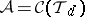shows the importance of the density topology in real analysis, since the classis strongly tied to the theory of Lebesgue integration and differentiation. For example, a bounded function is approximately continuous if and only if it is a derivative.

The topological properties of the density topology onare known quite well. Everyis Lebesgue measurable. The topology is connected, completely regular but not normal (cf. also Connected space; Completely-regular space; Normal space). A set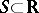is-nowhere dense if and only if it has Lebesgue measure zero (cf. Nowhere-dense set). Also,considered with the bitopological structure [a8] of the density and natural topologies is normal in the bitopological sense. (This is known as the Luzin–Menshov theorem [a1].)

The density topology onforis also defined from the notion of a density point. However, in this case there are different notions of the density point, depending on different neighbourhood bases at the point. For example, all points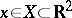satisfying the condition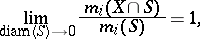where the setsare chosen among the squares centred at, are called ordinary density points of. This leads to the ordinary density topology on[a10]. Similarly, by choosing the setsfrom the family of all rectangles centred atwith sides parallel to the axes one obtains the strong density points [a10] and strong density topology. The ordinary density topology is completely regular, unlike the strong density topology [a4] (cf. also Completely-regular space). However, from the real analysis point of view, the strong density topology is usually more useful [a6].

A category analogue of the density topology, introduced by W. Wilczyński [a9], is called the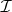-density topology. It is Hausdorff, but not regular (cf. Hausdorff space; Regular space). The weak topology generated by the class of all-approximately continuous functions is known as the deep-density topology. It is completely regular, but not normal (cf. Completely-regular space; Normal space).

Most of the topological information concerning the topologiesand its category analogues can be found in [a2]. This monograph contains an exhaustive study of sixteen different classes of continuous functions (fromto) that can be formed by putting the natural topology or either of these density topologies on the domain and the range.

How to Cite This Entry:
Density topology. Encyclopedia of Mathematics. URL: http://encyclopediaofmath.org/index.php?title=Density_topology&oldid=13331
This article was adapted from an original article by K. Ciesielski (originator), which appeared in Encyclopedia of Mathematics - ISBN 1402006098. See original article